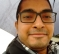## Frequency Shifting ConfusionStarted by 5 years ago10 replieslatest reply 5 years ago156 views

Hi,

It might be a very silly question. I have a baseband zigbee dump (zigbee._baseband.mat attached). I wish to shift it to -7 MHz, -2 MHz to the left and also 3 MHz, 8 MHz to the right. But when I multiply with corresponding carries and plot the fft, I see just opposite. What I am missing here?Here is the script

~~~~~~~~~~~~~~~~~~~~~~~~~~~~~~~~~~~~~~~~~~~~~~~~~~~~~~~~~~~~

close all

fc2430 = -7e6;

fc2435 = -2e6;

fc2440 = 3e6;

fc2445 = 8e6;

fs = 20e6;

t = (0:1/fs:((1/fs)*(length(interference)))-1/fs);

carrier2430 = exp(1i*2*pi*fc2430*t)'; % shift to -7 MHz

carrier2435 = exp(1i*2*pi*fc2435*t)'; % shift to -2 MHz

carrier2440 = exp(1i*2*pi*fc2440*t)'; % shift to 3 MHz

carrier2445 = exp(1i*2*pi*fc2445*t)'; % shift to 8 MHz

interference2430 = interference.*carrier2430;

interference2435 = interference.*carrier2435;

interference2440 = interference.*carrier2440;

interference2445 = interference.*carrier2445;

subplot(6,1,1)

plot([-64/2:64/2-1]*(fs)/64,(fftshift(abs(fft(interference,64)))))

subplot(6,1,2)

plot([-64/2:64/2-1]*(fs)/64,(fftshift(abs(fft(interference2430,64)))))

subplot(6,1,3)

plot([-64/2:64/2-1]*(fs)/64,(fftshift(abs(fft(interference2435,64)))))

subplot(6,1,4)

plot([-64/2:64/2-1]*(fs)/64,(fftshift(abs(fft(interference2440,64)))))

subplot(6,1,5)

plot([-64/2:64/2-1]*(fs)/64,(fftshift(abs(fft(interference2445,64)))))zigbee_baseband.mat

[ - ]Do you mean shift by -7 Mhz or shift to -7 Mhz? Becuase the plot isn't clear enough.

[ - ]As the baseband is it 0 MHz, either is applicable i.e. shifting to -7 MHz or shifting by -7 MHz.

[ - ]What it seems to be happening is that the spectrum is reversed, probably due to aliasing.

[ - ]What is "interference"? Could you plot without multiplying by it?

[ - ]Interference is zigbee. Yes when I plot it without multiplying with carrier, it lands on 0 MHz. The very first plot in the subplot.

[ - ]Why is there minus at -1/fs

t = (0:1/fs:((1/fs)*(zb_packet_len))-1/fs)
[ - ]So that the length of time array is equal to the length of interference. Apology these was a confusion in naming.

load('zigbee_baseband.mat') % Baseband  loads 'interference' variable to workspace. This is actually zigbee baseband oversampled at 20 MHz.

I just corrected in the post also.

[ - ]I notice the signal shape at baseband is slightly different when frequency shifted. This may give a clue

[ - ]